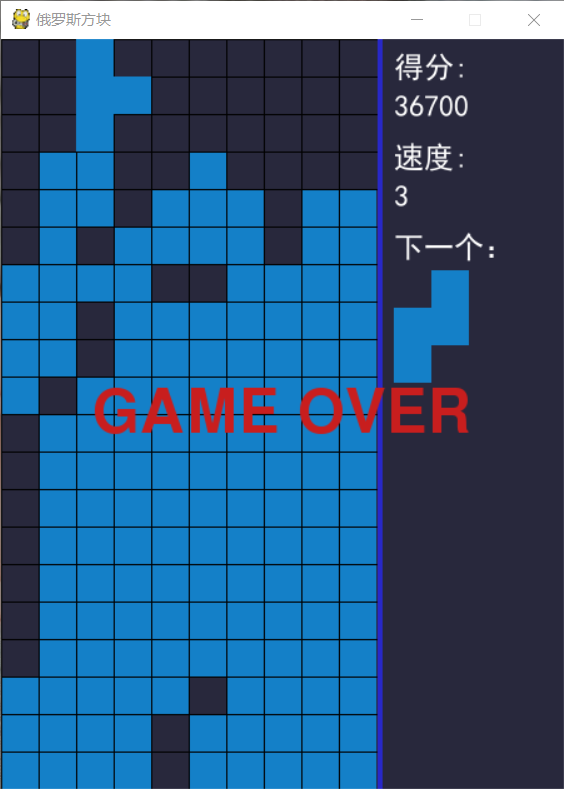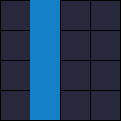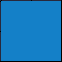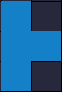# Python：游戏：300行代码实现俄罗斯方块## 外形

import sys
import pygame
from pygame.locals import *

SIZE = 30  # 每个小方格大小
BLOCK_HEIGHT = 20  # 游戏区高度
BLOCK_WIDTH = 10   # 游戏区宽度
BORDER_WIDTH = 4   # 游戏区边框宽度
BORDER_COLOR = (40, 40, 200)  # 游戏区边框颜色
SCREEN_WIDTH = SIZE * (BLOCK_WIDTH + 5)  # 游戏屏幕的宽
SCREEN_HEIGHT = SIZE * BLOCK_HEIGHT      # 游戏屏幕的高
BG_COLOR = (40, 40, 60)  # 背景色
BLACK = (0, 0, 0)

def print_text(screen, font, x, y, text, fcolor=(255, 255, 255)):
imgText = font.render(text, True, fcolor)
screen.blit(imgText, (x, y))

def main():
pygame.init()
screen = pygame.display.set_mode((SCREEN_WIDTH, SCREEN_HEIGHT))
pygame.display.set_caption('俄罗斯方块')

font1 = pygame.font.SysFont('SimHei', 24)  # 黑体24
font_pos_x = BLOCK_WIDTH * SIZE + BORDER_WIDTH + 10  # 右侧信息显示区域字体位置的X坐标
font1_height = int(font1.size('得分'))

score = 0           # 得分

while True:
for event in pygame.event.get():
if event.type == QUIT:
sys.exit()

# 填充背景色
screen.fill(BG_COLOR)
# 画游戏区域分隔线
pygame.draw.line(screen, BORDER_COLOR,
(SIZE * BLOCK_WIDTH + BORDER_WIDTH // 2, 0),
(SIZE * BLOCK_WIDTH + BORDER_WIDTH // 2, SCREEN_HEIGHT), BORDER_WIDTH)
# 画网格线 竖线
for x in range(BLOCK_WIDTH):
pygame.draw.line(screen, BLACK, (x * SIZE, 0), (x * SIZE, SCREEN_HEIGHT), 1)
# 画网格线 横线
for y in range(BLOCK_HEIGHT):
pygame.draw.line(screen, BLACK, (0, y * SIZE), (BLOCK_WIDTH * SIZE, y * SIZE), 1)

print_text(screen, font1, font_pos_x, 10, f'得分: ')
print_text(screen, font1, font_pos_x, 10 + font1_height + 6, f'{score}')
print_text(screen, font1, font_pos_x, 20 + (font1_height + 6) * 2, f'速度: ')
print_text(screen, font1, font_pos_x, 20 + (font1_height + 6) * 3, f'{score // 10000}')
print_text(screen, font1, font_pos_x, 30 + (font1_height + 6) * 4, f'下一个：')

pygame.display.flip()

if __name__ == '__main__':
main()


## 方块I 型O 型T 型S 型Z 型L 型J 型

['.0..',
'.0..',
'.0..',
'.0..']


['....',
'....',
'0000',
'....']['.0..',
'.0..',
'.0..',
'.0..']


['.0.',
'000',
'...']


game_area = [['.'] * BLOCK_WIDTH for _ in range(BLOCK_HEIGHT)]


cur_block = None   # 当前下落方块
cur_pos_x, cur_pos_y = 0, 0  # 当前下落方块的坐标


from collections import namedtuple

Point = namedtuple('Point', 'X Y')
Block = namedtuple('Block', 'template start_pos end_pos name next')

# S形方块
S_BLOCK = [Block(['.00',
'00.',
'...'], Point(0, 0), Point(2, 1), 'S', 1),
Block(['0..',
'00.',
'.0.'], Point(0, 0), Point(1, 2), 'S', 0)]


BLOCKS = {'O': O_BLOCK,
'I': I_BLOCK,
'Z': Z_BLOCK,
'T': T_BLOCK,
'L': L_BLOCK,
'S': S_BLOCK,
'J': J_BLOCK}

def get_block():
block_name = random.choice('OIZTLSJ')
b = BLOCKS[block_name]
idx = random.randint(0, len(b) - 1)
return b[idx]

# 获取旋转后的方块
def get_next_block(block):
b = BLOCKS[block.name]
return b[block.next]


def _judge(pos_x, pos_y, block):
nonlocal game_area
for _i in range(block.start_pos.Y, block.end_pos.Y + 1):
if pos_y + block.end_pos.Y >= BLOCK_HEIGHT:
return False
for _j in range(block.start_pos.X, block.end_pos.X + 1):
if pos_y + _i >= 0 and block.template[_i][_j] != '.' and game_area[pos_y + _i][pos_x + _j] != '.':
return False
return True


## 停靠

def _dock():
nonlocal cur_block, next_block, game_area, cur_pos_x, cur_pos_y, game_over
for _i in range(cur_block.start_pos.Y, cur_block.end_pos.Y + 1):
for _j in range(cur_block.start_pos.X, cur_block.end_pos.X + 1):
if cur_block.template[_i][_j] != '.':
game_area[cur_pos_y + _i][cur_pos_x + _j] = '0'
if cur_pos_y + cur_block.start_pos.Y <= 0:
game_over = True
else:
# 计算消除
remove_idxs = []
for _i in range(cur_block.start_pos.Y, cur_block.end_pos.Y + 1):
if all(_x == '0' for _x in game_area[cur_pos_y + _i]):
remove_idxs.append(cur_pos_y + _i)
if remove_idxs:
# 消除
_i = _j = remove_idxs[-1]
while _i >= 0:
while _j in remove_idxs:
_j -= 1
if _j < 0:
game_area[_i] = ['.'] * BLOCK_WIDTH
else:
game_area[_i] = game_area[_j]
_i -= 1
_j -= 1
cur_block = next_block
next_block = blocks.get_block()
cur_pos_x, cur_pos_y = (BLOCK_WIDTH - cur_block.end_pos.X - 1) // 2, -1 - cur_block.end_pos.Y


Python：游戏：贪吃蛇

Python：游戏：扫雷（附源码）

Python：游戏：五子棋之人机对战

### 扫码关注我的个人公众号，后台回复 “俄罗斯方块” 获取源码。